Ex 1.2

Chapter 1 Class 10 Real Numbers
Serial order wise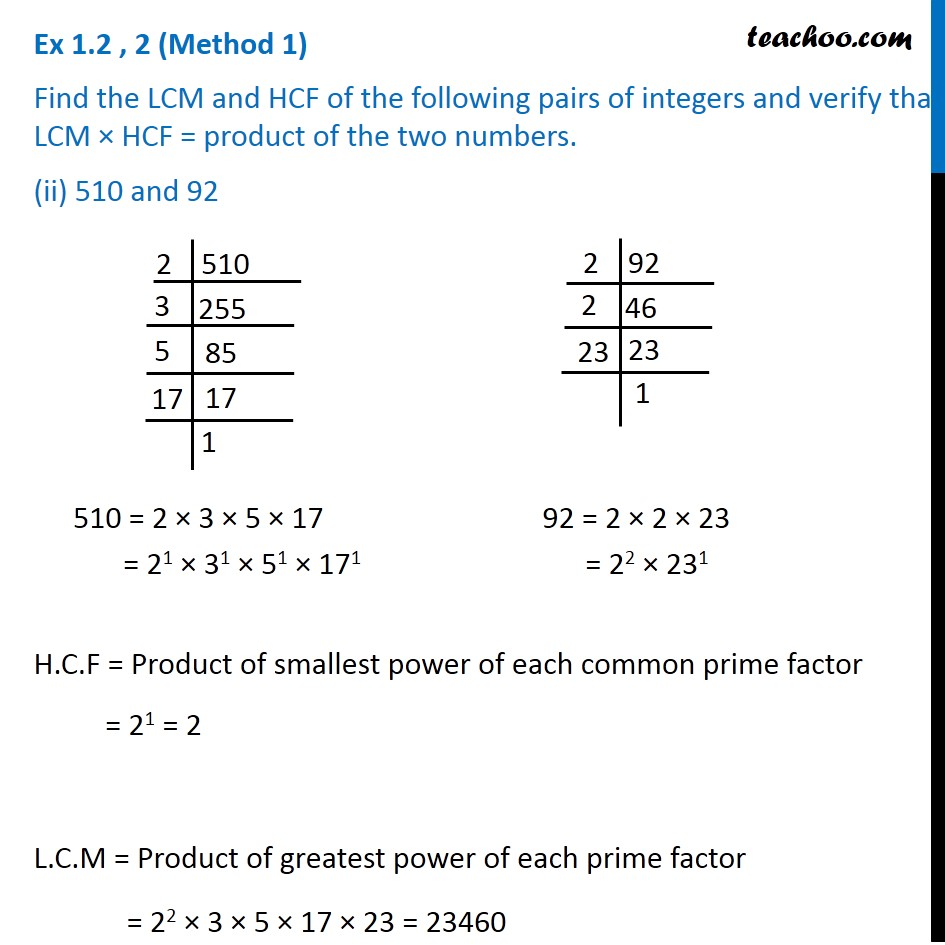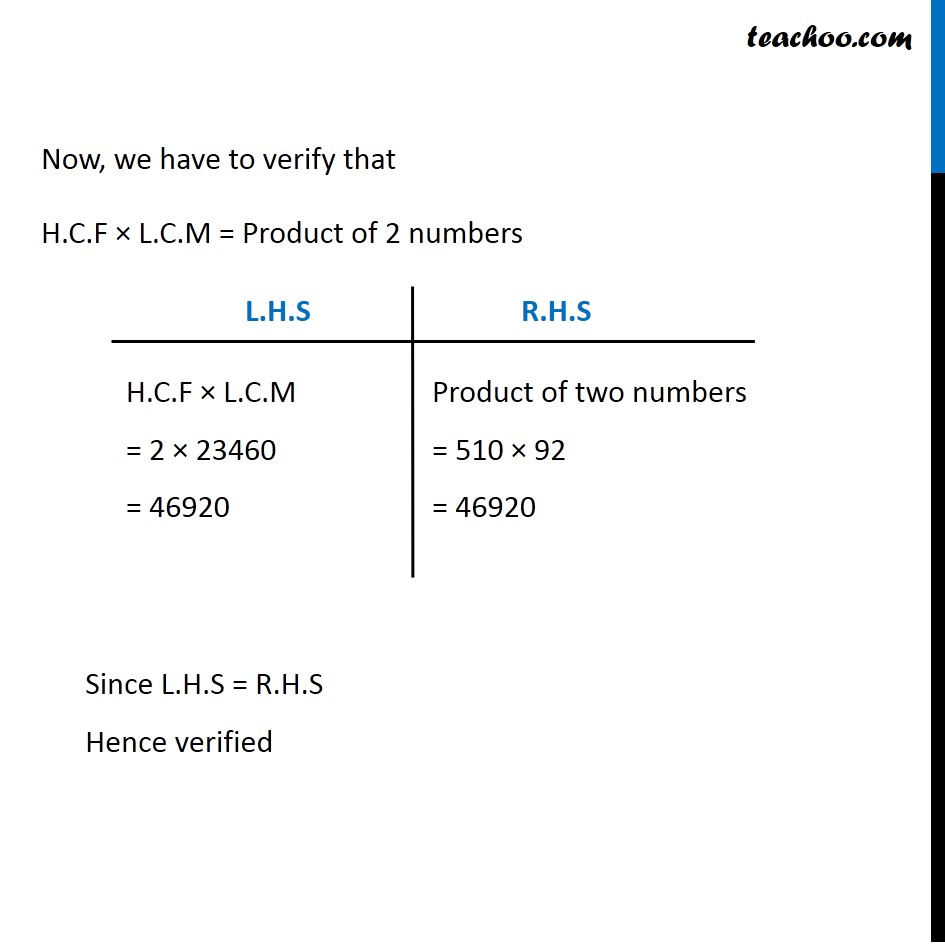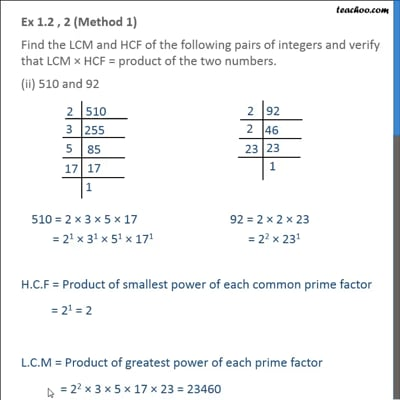This video is only available for Teachoo black users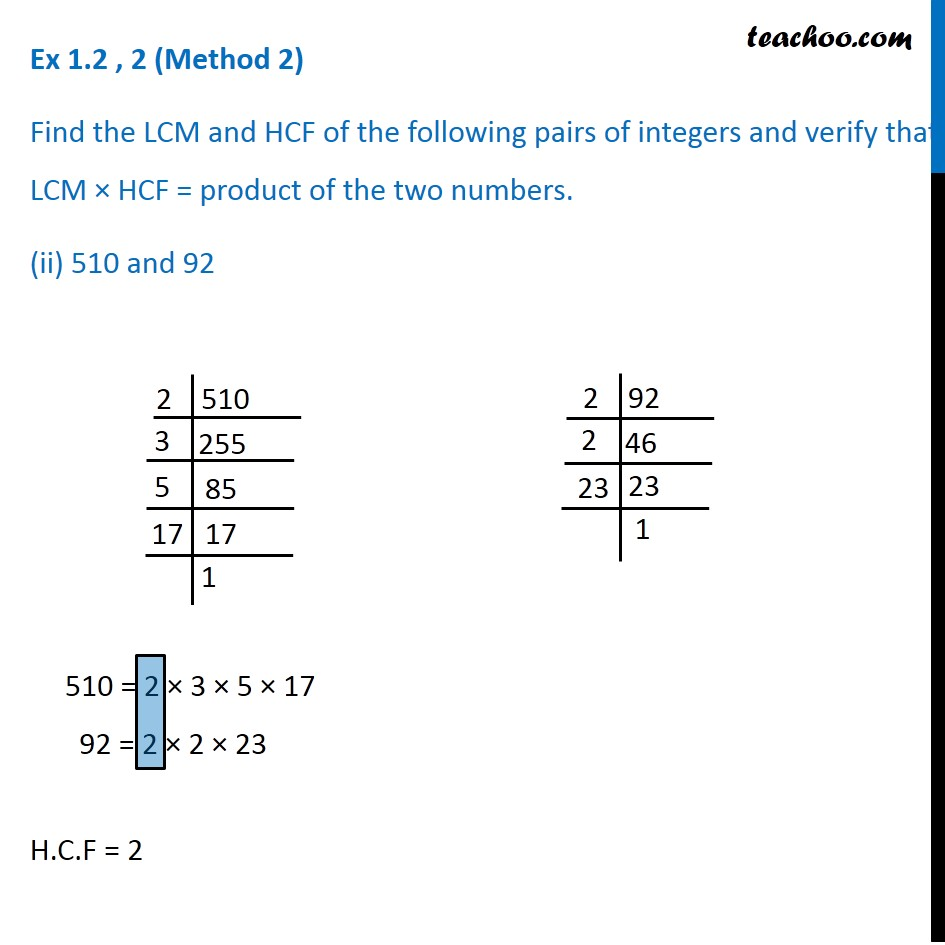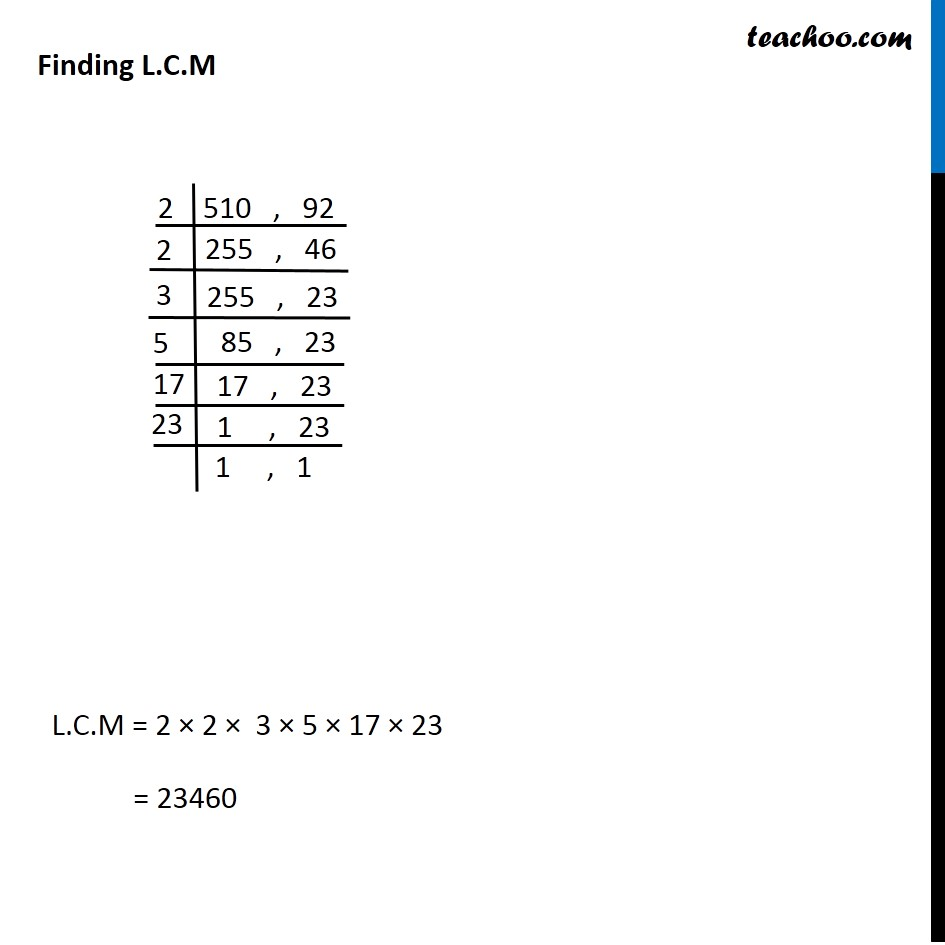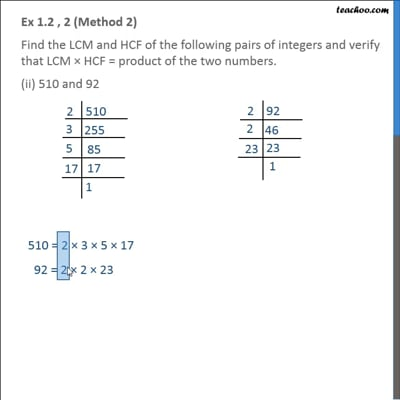This video is only available for Teachoo black users

Introducing your new favourite teacher - Teachoo Black, at only ₹83 per month

### Transcript

Ex 1.2 , 2 (Method 1) Find the LCM and HCF of the following pairs of integers and verify that LCM × HCF = product of the two numbers. (ii) 510 and 92 510 = 2 × 3 × 5 × 17 = 21 × 31 × 51 × 171 92 = 2 × 2 × 23 = 22 × 231 H.C.F = Product of smallest power of each common prime factor = 21 = 2 L.C.M = Product of greatest power of each prime factor = 22 × 3 × 5 × 17 × 23 = 23460 Now, we have to verify that H.C.F × L.C.M = Product of 2 numbers H.C.F × L.C.M = 2 × 23460 = 46920 Product of two numbers = 510 × 92 = 46920 Since L.H.S = R.H.S Hence verified Ex 1.2 , 2 (Method 2) Find the LCM and HCF of the following pairs of integers and verify that LCM × HCF = product of the two numbers. (ii) 510 and 92 510 = 2 × 3 × 5 × 17 92 = 2 × 2 × 23 H.C.F = 2 Finding L.C.M L.C.M = 2 × 2 × 3 × 5 × 17 × 23 = 23460 Now, we have to verify that H.C.F × L.C.M = Product of 2 numbers H.C.F × L.C.M = 2 × 23460 = 46920 Product of two numbers = 510 × 92 = 46920 Since L.H.S = R.H.S Hence verified